## Friday, 27 January 2012

### SHAPE II. Basic shapes

Basic Shapes_____________________________________________
The 3 basic shapes are:

1.Circle: is a simple shape, a closed plane curve. All points are the same distance from the center.
• The distance between any of the points and the centre is called the radius.
• The Diameter starts at one side of the circle, goes through the center and ends on the other side.
2. Triangle: is a polygon.
3. Square: is a polygon.
Polygons_____________________________________________________
• A polygon is a flat shape with straight sides. The points where two edges meet are the polygon's vertices (singular: vertex) or corners.
• Polygons are 2-dimensional shapes. They are made of straight lines, and the shape is "closed" (all the lines connect up).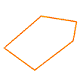Polygon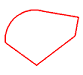Not a polygon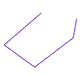Not a polygon
If there is a curve o the shape is not closed it is not a polygon.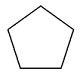Regular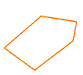Irregular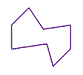irregular
There are regular and irregular polygons.A regular polygon is a polygon whose sides are all the same length, and whose angles are all the same.

Exercise______________________________________________________

1. Make the students draw shapes out of the three basic ones: circle, triangle and square.